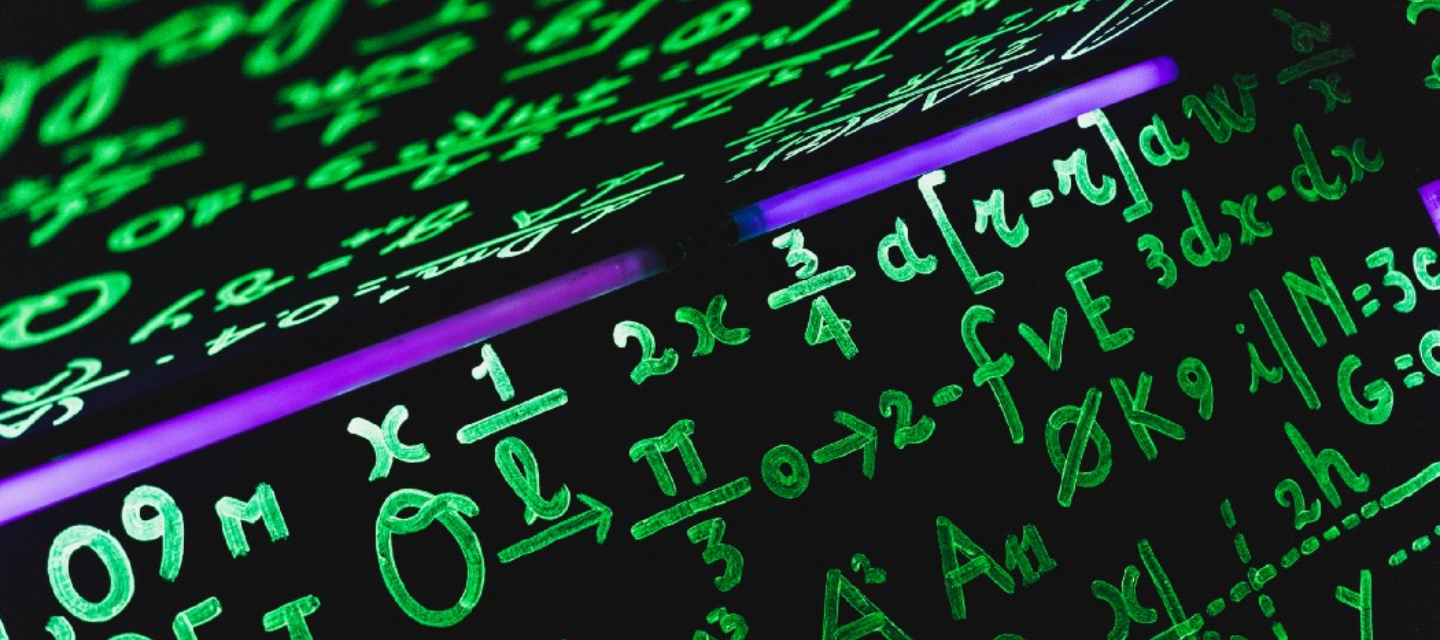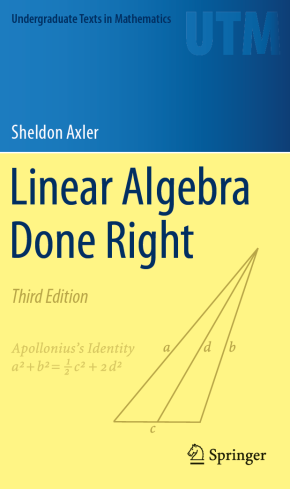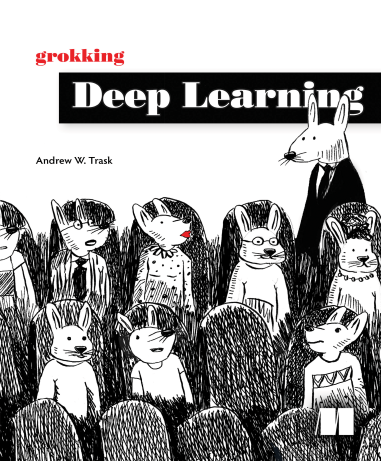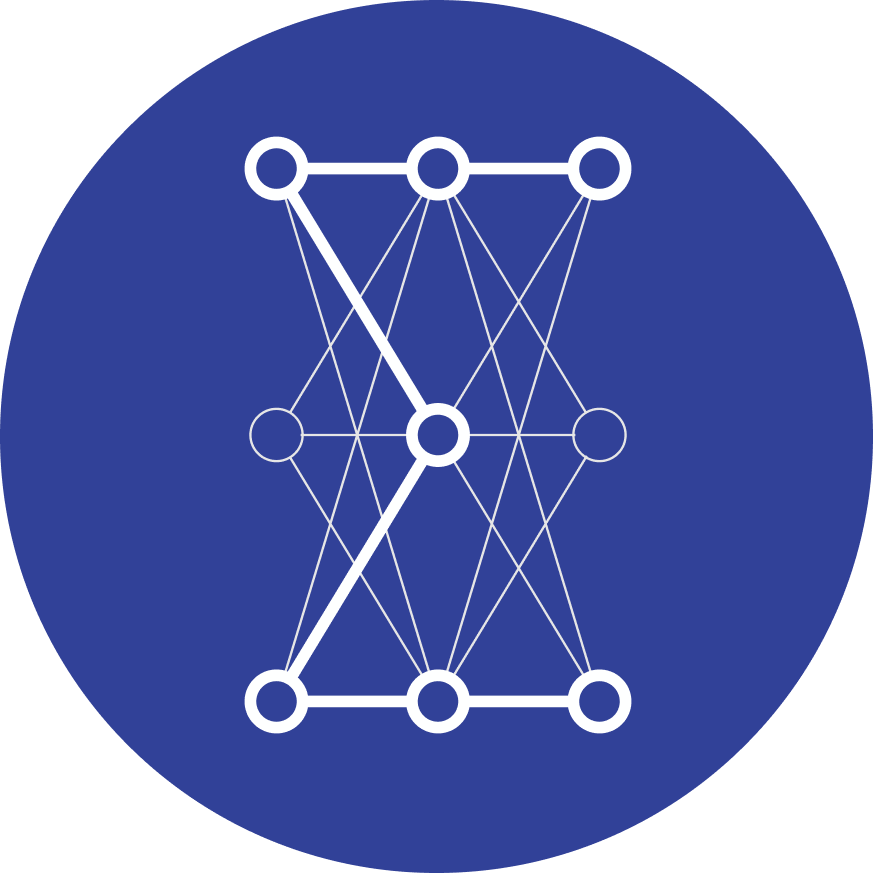# 5 Books That Will Teach You the Math Behind Machine LearningAfter the explosive growth of open-source machine learning and deep learning frameworks, the field is more accessible than ever. Thanks to this, it went from a tool for researchers to a widely adopted and used method, fueling the insane growth of technology we experience now. Understanding how algorithms work behind the hood can give you a huge advantage in designing, developing, and debugging machine learning systems. Due to its mathematical nature, this task can seem daunting for many. However, this does not have to be the way.

From a high level, there are four pillars of mathematics in machine learning:

1. linear algebra
2. probability theory
3. multivariate calculus
4. optimization.

It takes time to build a solid foundation of these. Understanding the inner workings of machine learning won’t be an afternoon project. But given that you consistently dedicate time for this, you can go pretty far in a short amount of time. There are some great resources to guide you along the way. In this post, I have selected five that will be an excellent guide for you.

## Linear Algebra Done Right by Sheldon AxlerLinear algebra is a beautiful but challenging subject for beginners if it is taught the “classical” way, which is determinants and matrices first, vector spaces later. However, when it is done the other way around, it is surprisingly intuitive and clear. This book presents linear algebra in a friendly and insightful way. I wish I had learned it from this book instead of the old way.

You can find the author’s page about the book here.

## Multivariate Calculus by Denis Auroux (from MIT OpenCourseWare)

I have cheated a little bit here since this is not a book but an actual university course on multivariate calculus at MIT, recorded and made available for the public. Out of all the resources I know, this is the best introduction to the subject by far. It doesn’t hurt to have a background in univariate calculus, but we can follow the lectures without it.

You can find the entire course here.

One thing this course doesn’t cover well is the gradient descent algorithm, which is fundamental for neural networks. If you want to learn more about this, I wrote an introductory post on the subject, which explains gradient descent from scratch.

## Grokking Deep Learning by Andrew TraskThis book is one of my favorite machine learning books in general.

It contains a complete hands-on introduction to the inner workings of neural networks, with code snippets covering all of the material. Even though not explicitly geared towards advanced mathematics, you’ll know more about the mathematics of deep learning than 95% of data scientists, machine learning engineers, and other developers by the end of this book.

You’ll also build a neural network from scratch, which is probably the best learning exercise you can undertake.

## Deep Learning by Ian Goodfellow, Yoshua Bengio, and Aaron Courville

This is where all the topics you have learned come together. It was written by some of the greatest minds in machine learning. This book synthesizes the mathematical theory and puts the heavy machinery into use, providing a solid guide to state-of-the-art deep learning methods such as convolutional and recurrent networks, autoencoders, and many more.

The best is that this book is freely available online for everyone. This is the number one resource for deep learning researchers and developers, so this is pretty great.

Among all the resources I have listed here, this is probably the most difficult to read. Understanding deep learning requires looking at the algorithms from a probabilistic perspective, which can be difficult. If you would like to learn how we can translate a problem into the language of probability and statistics, I have written a detailed guide for you, where I explain the essential details in a beginner-friendly way.

## Mathematics of Machine Learning by Tivadar DankaTrust me, I am familiar with the steep learning curve of mathematics for machine learning. Especially if you have a software engineering background, it is hard to find a comprehensive resource that is written with the applications in mind instead of piling complex concepts on top of each other.

Thus, I have decided to write one that takes the readers from high school mathematics to state-of-the-art machine learning, focusing on clear and intuitive explanations. I am working to create the best resource to study the mathematics of machine learning out there. I cover every topic that you might need, with a focus on

• linear algebra,
• multivariable calculus,
• probability theory,
• and the internals of neural networks.

The book is currently out in early access! You can find the details here if you are interested!

## Let’s get to learning!

As I have mentioned, you probably won’t be able to burn through all these resources in an afternoon. You’ll need to work hard, but it will pay off in the future. Building up knowledge is the best investment. In the end, this will give you a huge advantage in building machine learning systems. (Not to mention that the theory behind machine learning is beautiful.)